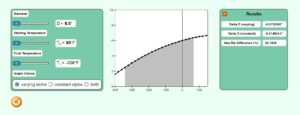# Chapter 01 Simulating Engineering Modeling Errors

Description:  This simulation depicts the difference between the outputs by using two different models of solving the problem of how much a metal contract when subjected to a large temperature change.Keywords:  modeling, engineering modeling, thermal expansion, thermal contraction, coefficient of thermal expansion.

Learning Objectives:  After successful completion of running the simulation with proper questions asked by the instructor, the student would be able to 1) note the calculation of the contraction in metal by modeling the variation of coefficient of thermal expansion as a function of temperature 2) note the calculation of the contraction in metal by modeling coefficient of thermal expansion as a constant function of temperature 3) note the differences between the two, 4) recognize the need for numerical methods for more accurate solutions.

Full Resources:  The full resources for the topic of the modeling differences are given here.